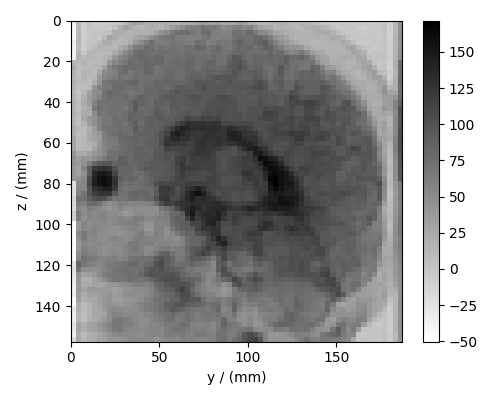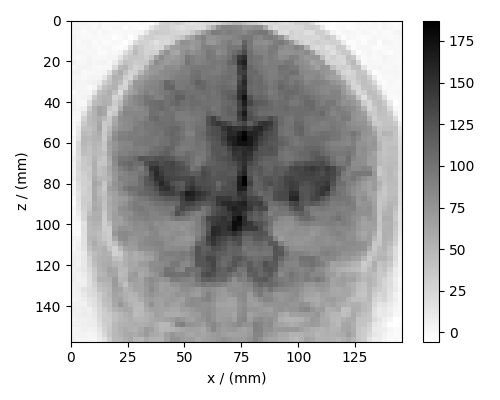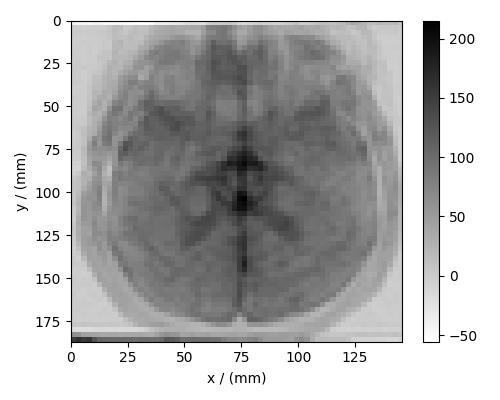# Diffusion tensor MRI, 3D{6} dataset¶

The following is an example of a 3D{6} diffusion tensor MRI dataset with three spatial dimensions, $$d=3$$, and one, $$p=1$$, dependent-variable with six components. For illustration, we have reduced the size of the dataset. The complete diffusion tensor MRI dataset, in the CSDM format, is available online. The original dataset 1 is also available.

Let’s import the CSDM data-file and look at its data structure.

import csdmpy as cp

domain = "https://www.ssnmr.org/sites/default/files/CSDM"
filename = f"{domain}/tensor/human_brain/brain_MRI_reduced_example.csdf"


There are three linear dimensions in this dataset, corresponding to the x, y, and z spatial dimensions,

x = diff_mri.dimensions
print(x.label, x.label, x.label)

x y z


and one six-component dependent variables holding the diffusion tensor components. Because the diffusion tensor is a symmetric second-rank tensor, we only need six tensor components. The components of the tensor are ordered as

y = diff_mri.dependent_variables
print(y.component_labels)

['dxx', 'dxy', 'dxz', 'dyy', 'dyz', 'dzz']


The symmetric matrix information is also found with the quantity_type attribute,

print(y.quantity_type)

symmetric_matrix_3


which implies a 3x3 symmetric matrix.

Visualize the dataset

In the following, we visualize the isotropic diffusion coefficient, that is, the average of the $$d_{xx}$$, $$d_{yy}$$, and $$d_{zz}$$ tensor components. Since it’s a three-dimensional dataset, we’ll visualize the projections onto the three dimensions.

# the isotropic diffusion coefficient.
# component at index 0 = dxx
# component at index 3 = dyy
# component at index 5 = dzz
isotropic_diffusion = (y.components + y.components + y.components) / 3


In the following, we use certain features of the csdmpy module. Please refer to Generating CSDM objects for further details.

# Create a new csdm object from the isotropic diffusion coefficient array.
new_csdm = cp.as_csdm(isotropic_diffusion, quantity_type="scalar")

# Add the dimensions from diff_mri object to the new_csdm object.
for i, dim in enumerate(x):
new_csdm.dimensions[i] = dim


Now, we can plot the projections of the isotropic diffusion coefficients along the respective dimensions as

import matplotlib.pyplot as plt

# projection along the x-axis.
plt.figure(figsize=(5, 4))
ax = plt.subplot(projection="csdm")
cb = ax.imshow(new_csdm.sum(axis=0), cmap="gray_r", origin="upper", aspect="auto")
plt.colorbar(cb, ax=ax)
plt.tight_layout()
plt.show()# projection along the y-axis.
plt.figure(figsize=(5, 4))
ax = plt.subplot(projection="csdm")
cb = ax.imshow(new_csdm.sum(axis=1), cmap="gray_r", origin="upper", aspect="auto")
plt.colorbar(cb, ax=ax)
plt.tight_layout()
plt.show()# projection along the z-axis.
plt.figure(figsize=(5, 4))
ax = plt.subplot(projection="csdm")
cb = ax.imshow(new_csdm.sum(axis=2), cmap="gray_r", origin="upper", aspect="auto")
plt.colorbar(cb, ax=ax)
plt.tight_layout()
plt.show()Citation

1

Diffusion tensor MRI data; 2000.

Total running time of the script: ( 0 minutes 0.969 seconds)

Gallery generated by Sphinx-Gallery